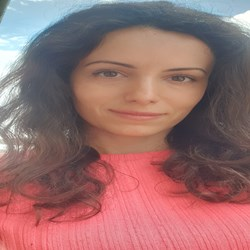+ Нов въпросGalinagg 3 Точки

## Kamino Factory - Exercise, Java

Колеги, моля помогнете, къде греша ? Всичко излиза в IntelliJ, но не и в Judge :(((((

Задача 09. Kamino Factory

https://judge.softuni.bg/Contests/Compete/Index/1247#0

Моето решение:

https://pastebin.com/XyY9Nndc

Тагове:
0
25/02/2021 13:09:45Axiomatik 2054 Точки

Don't have access to that competition, but hope that this rewritte works - otherwise there are plenty of working JAVA solutions in the forum with which you can certainly find the last errors (search "Kamino"). Usually, if you have a running code with this exercise, it's the final validations for the best sequence that cause a problem.

Best,

``````import java.util.Arrays;
import java.util.Scanner;

public class demo {
public static void main(String[] args) {
Scanner scanner = new Scanner(System.in);

int length = Integer.parseInt(scanner.nextLine());
String numbers = scanner.nextLine();

int combinationCount = 0;
int counterCoincidence = 1;

int baseMaxCount = 0;
int baseIndex = 0;
int sumElementsBase = 0;
int baseCombination = 0;
int[] baseArray = new int[length];

while (!numbers.equals("Clone them!")) {
int[] arr = Arrays.stream((numbers).split("!")).mapToInt(e -> Integer.parseInt(e)).toArray();
combinationCount++;

int maxCount = 0;
int index1 = 0;
int sumElements = 0;
int firstIndex = -1;

for (int index = 0; index < arr.length - 1; index++) {
if (arr[index] == arr[index + 1] && arr[index] == 1) {
counterCoincidence++;
if(firstIndex == -1){
firstIndex = index;
}
// for (int i = 0; i <= arr.length - 1; i++) {
//     if (arr[i] == 1) {
//         sumElements++;
//     }
// }
//if (counterCoincidence > maxCount) {
//    maxCount = counterCoincidence;
//    index1 = index;
//}
}
//else {
//    counterCoincidence = 1;
//}
}

for (int i = 0; i <= arr.length - 1; i++) {
if (arr[i] == 1) {
sumElements++;
}
}
// You should select the sequence with the longest subsequence of ones.
if (counterCoincidence > baseMaxCount) {
baseArray = arr;
baseMaxCount = counterCoincidence;
baseIndex = firstIndex;
sumElementsBase = sumElements;
baseCombination = combinationCount;
}
// If there are several sequences with same length of subsequence of ones,
// print the one with the leftmost starting index
if (counterCoincidence == baseMaxCount && firstIndex < baseIndex){
baseArray = arr;
baseMaxCount = counterCoincidence;
baseIndex = firstIndex;
baseCombination = combinationCount;
sumElementsBase = sumElements;
}
// if there are several sequences with same length and starting index,
// select the sequence with the greater sum of its elements
if(counterCoincidence == baseMaxCount && firstIndex == baseIndex && sumElements > sumElementsBase){
baseArray = arr;
baseMaxCount = counterCoincidence;
baseIndex = firstIndex;
baseCombination = combinationCount;
sumElementsBase = sumElements;
}

numbers = scanner.nextLine();
}

System.out.printf("Best DNA sample %d with sum: %d.%n", baseCombination, sumElementsBase);
for (int i = 0; i <= baseArray.length - 1; i++) {
System.out.print(baseArray[i] + " ");
}
}
}
``````

0
25/02/2021 18:00:47
Можем ли да използваме бисквитки?
Ние използваме бисквитки и подобни технологии, за да предоставим нашите услуги. Можете да се съгласите с всички или част от тях.
Назад
Функционални
Използваме бисквитки и подобни технологии, за да предоставим нашите услуги. Използваме „сесийни“ бисквитки, за да Ви идентифицираме временно. Те се пазят само по време на активната употреба на услугите ни. След излизане от приложението, затваряне на браузъра или мобилното устройство, данните се трият. Използваме бисквитки, за да предоставим опцията „Запомни Ме“, която Ви позволява да използвате нашите услуги без да предоставяте потребителско име и парола. Допълнително е възможно да използваме бисквитки за да съхраняваме различни малки настройки, като избор на езика, позиции на менюта и персонализирано съдържание. Използваме бисквитки и за измерване на маркетинговите ни усилия.
Рекламни
Използваме бисквитки, за да измерваме маркетинг ефективността ни, броене на посещения, както и за проследяването дали дадено електронно писмо е било отворено.